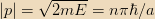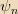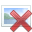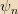# Particle in a box - Momentum and Energy

Hi,

I have a problem understanding the particle in a box (V=0 inside, V=∞ outside), how is it possible that momentum can vary continuously while the energy spectrum is discrete? Aren't they related by E=p2/2m? What I am missing? Thanks!

This is one of the key "weirdnesses" of quantum mechanics. When we build a Hamiltonian to specify a system, we take a classical standpoint - the formula $E=\frac{p^2}{2m}$ is inherently classical since $$p=mv\implies\frac{p^2}{2m}=\frac{m^2v^2}{2m} = \frac{1}{2}mv^2 = E_{free}.$$ Indeed, for a free quantum particle the energy spectrum is continuous, since the Hamiltonian is an analytic function of momentum and thus has the same eigenstates with different eigenvalues.

However, the Hamiltonian for a particle in a box $$\mathcal H = \begin{cases}\frac{p^2}{2m}&\text{if }0\leq x\leq L\\\infty&\text{otherwise}\end{cases}$$ is definitely NOT an analytic function of momentum. When this happens, the theorem that the eigenstates are the same is no longer applicable, and thus you get no more guarantee that the quantum energy levels will be the same as the classical ones. In particular, a continuous spectrum versus a discrete one is then possible.

Another example: the Hamiltonian for a harmonic oscillator is $$\mathcal H = \frac{p^2+(m\omega x)^2}{2m}.$$ This is a function of both the momentum and position operators, and thus is not purely an analytic function of either. This implies the same (lack of) results about eigenstates as above.

Orodruin
Staff Emeritus
Homework Helper
Gold Member
It is actually even worse, the momentum eigenstates are not even part of the appropriate Hilbert space that describes the particle in the box. As such, momentum cannot be measured without changing the system itself.

If you instead take a finite potential well, you will soon realise that the Hamiltonian and the momentum operators do not commute and as a result there is no common set of eigenstates.

Simon Bridge
Homework Helper
The classical relationship between energy and momentum is an emergent property of the quantum statistics - it is not absolute.
The relation you are missing is: <E> = <p>2/2m where <X> indicates the expectation value of X.

Orodruin
Staff Emeritus
Homework Helper
Gold Member
<E> = <p>^2/2m
This is simply wrong, <p> = 0 for all eigenstates of the Hamiltonian in the case of the infinite potential well. Since H = p^2/(2m) + V, it follows that <E> = <p^2>/(2m) + <V>. For all states of the infinite potential well, <V> = 0, so <E> = <p^2>/(2m).

Simon Bridge
Homework Helper
Oh typo ... <p^2>/2m, thanks.

Staff Emeritus
This is simply wrong, <p> = 0 for all eigenstates of the Hamiltonian in the case of the infinite potential well.

As is required by the symmetry of the system. In a stationary state, the system must spend as much time with p to the left as p to the right, so <p>=0.

I think that my question is wrong, the momentum is quantized actually.

En=n2π2ħ2/2mL2
Pn2=2mEn=2mn2π2ħ2/2mL2
Pn=±nπħ/L

Last edited:
vanhees71
Gold Member
It is actually even worse, the momentum eigenstates are not even part of the appropriate Hilbert space that describes the particle in the box. As such, momentum cannot be measured without changing the system itself.

If you instead take a finite potential well, you will soon realise that the Hamiltonian and the momentum operators do not commute and as a result there is no common set of eigenstates.
To state it even more drastically: There is no such thing as momentum for a particle in a finite potential pot with infinitely large wells. It simply doesn't make sense. There is of course a complete set of energy eigenvectors, and the energy spectrum is entirely discrete.

In reality, there's of course no such thing as infinitely high potential wells. It's just a simplified model system for a particle in a very deep potential well.

Staff Emeritus
I think that my question is wrong, the momentum is quantized actually.

The +/- there doesn't bother you? It should.

Orodruin
Staff Emeritus
Homework Helper
Gold Member
I think that my question is wrong, the momentum is quantized actually.

En=n2π2ħ2/2mL2
Pn2=2mEn=2mn2π2ħ2/2mL2
Pn=±nπħ/L

I suggest reading posts #3 and #9 above.

vanhees71
Gold Member
Again, there is no momentum operator and thus no momentum eigenfunctions. There are eigenfunctions and eigenvalues for the Hamiltonian:
$$-\frac{\hbar^2}{2m} u_E''(x)=E u_E(x).$$
This has to be solved with the boundary conditions ##u_E(\pm L/2)=0##. The solutions are of the form
$$u_E(x)=A \sin(k x), \quad u_E(x)=A \cos(k x).$$
$$k L/2=n \pi, \quad k L/2=(2n+1) \pi/2,$$
or
$$k_j=\frac{j \pi}{L}, \quad j \in \mathbb{N}=\{1,2,3,\ldots \}.$$
The energy eigenvalues are
$$E_j=\frac{\hbar^2 k_j^2}{2m}.$$
The eigenfunctions are
$$u_j(x)=A \begin{cases} \cos(k_j x) \quad \text{for} \quad j=2n+1, \quad n \in \{0,1,\ldots\},\\ \sin(k_j x) \quad \text{for} \quad j=2n, \quad n \in \{1,2,\ldots \}. \end{cases}$$
Note that these are NOT eigenfunctions of the would-be momentum operator, ##-\mathrm{i} \hbar \mathrm{d}_x##.

No. Take a look at the momentum-space wave functions ##\phi_n(p)## for the infinite square well (particle in a box):

http://physicspages.com/2012/10/04/infinite-square-well-momentum-space-wave-functions/

I found a page in the same site that proves my point:

"the momentum is equally likely to be in either direction. The magnitude of the momentum is a constant, since this is a state with fixed energy, and"

http://physicspages.com/2012/09/13/infinite-square-well-momentum/

Last edited:
Staff Emeritus
It's talking about the mean momentum, not about what you would get when you measure p. If you go to that page and up a bit, it will say "Thusis not an eigenfunction of momentum, since the momentum operator doesn’t yield the original wave function multiplied by a constant."

The +/- should have been a clue. If I am in an Eigenstate of energy, and E and p commute, I should have one and only one value of momentum. This means I have at least two. So E and p don't commute, and I am not in a momentum eigenstate.

jtbell
Mentor
The magnitude of the momentum is a constant, since this is a state with fixed energy, and"

This is wrong. If this were true, then the momentum space wave function for the n'th energy eigenstate would be basically a pair of Dirac delta functions, with the "spikes" at ##p = \pm n \pi \hbar / a##. This is clearly not true for the n = 1 and n = 2 states, whose graphs are on the page that I linked to. Those graphs use the variable ##\rho = pa/\hbar##, so the "spikes" on those graphs would be at ##\rho = \pm n\pi##.

Note that the graph for n = 2 does have maxima at or near ##\rho = \pm 2\pi##. I once did graphs for some higher values of n, which illustrated that the peaks become sharper as n increases.

A sinusoidal wave function corresponds to an exact value of p only if it extends to infinity in both directions. A sinusoidal wave function that is "chopped off" to zero at the sides of the well, corresponds to a sum (actually an integral: a Fourier integral) of infinitely many infinitely-long waves with different wavelengths and momenta. These waves have amplitudes such that they add up to zero outside the well.

It's talking about the mean momentum, not about what you would get when you measure p. If you go to that page and up a bit, it will say "Thusis not an eigenfunction of momentum, since the momentum operator doesn’t yield the original wave function multiplied by a constant."

OK, it's an eigenfunction of p2, isn't it?

ψn=(√2/a) sin (nπx/a)
(d2/dx2) sin (nπx/a) = -(nπ/a)2 sin (nπx/a)
p2ψn=-(iħ)2 (nπ/a)2ψn=(nπħ/a)2ψn

Last edited: